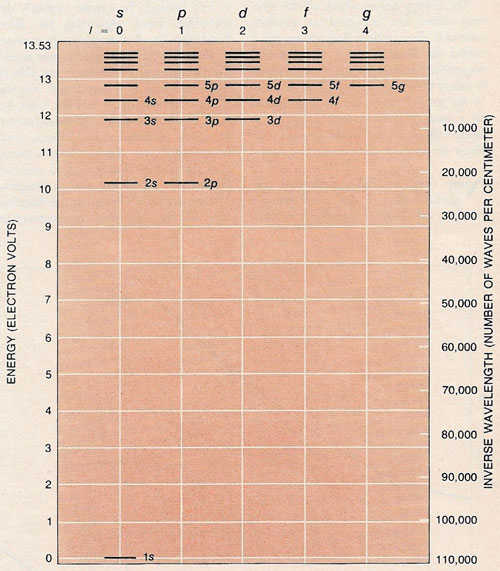A# electron configurationElectronic structure of an atom is represented by the energy levels its electrons can occupy. According to quantum theory the levels can assume only discrete values. The electronic structure shown here is for the hydrogen atom. As the energy is raised the permitted levels lie closer and closer together. When the electron's energy exceeds 13.53 V, however, the electron can no longer be held by the positive charge on the hydrogen's nucleus and escapes. As the angular momentum, l, of the electron increases in integral steps the minimum energy jumps upward one level at a time. In falling from the highest level to zero energy an electron emits ultraviolet radiation with a wavelength of 916 angstroms, which corresponds to not quite 110,000 waves/cm, as shown in the scale at right.

The electron configuration is the disposition of electrons among the orbitals of an atom. The electron configuration of the outer shells, in particular, is a key determinant of the chemical properties of the atom.

Each electron in an atom is associated with four characteristic quantum numbers, which are interrelated. These are:

· Principal quantum number, n (= 1, 2, 3, ...), which denotes the overall size and energy of an electron shell.
· Azimuthal quantum number, l (= 0, 1, 2, ..., n-1), which denotes the angular momentum and shape of an orbital. Azimuthal quantum numbers 0, 1, 2, and 3 correspond with the s, p, d, and f orbitals, respectively.
· Magnetic quantum number, m (= -l, -l+1, ... 0, ... l-1, l), which denotes the orientation of an orbital.
· Spin quantum number, s (= ± ½)

## s, p, d, and f orbitals

An s orbital is spherically symmetric and can hold a maximum of two electrons with opposite spins. A p orbital has a solid figure-of-eight shape. There are three equivalent p orbitals for each principal quantum number, corresponding to the three axes of rectangular coordinates.

The d and f orbitals are more complicated in shape. There are five equivalent d orbitals and seven equivalent f orbitals for each principal quantum number. Each orbital contains at most two electrons with opposite spins.

For principal quantum number 1 there are only s orbitals; for n = 2 there are only s and p orbitals; for n = 3 there are only s, p, and d orbitals; for higher values of n there are s, p, d, and f orbitals.

To obtain the electron configuration of an atom the appropriate number of electrons is placed in the orbitals in order of energy, the orbitals of lower energy being filled first (a rule called Aufbau's principle), subject to the proviso that for a set of equivalent orbitals – say the three p orbitals in a set – the electrons are placed one in each orbital until all the orbitals are half-filled (Hund's rule). The order of increasing energy for most atoms is 1s, 2s, 2p, 3s, 3p, 4s, 3d, 4p, 5s, 4d, 5p, 6s, 4f, 5d, 6p, 7s, ... In any atom no two electrons may have all quantum numbers the same (Pauli exclusion principle).

## Molecules

The electron configuration of molecules can be built up by direct addition of atomic orbitals (LCAO method) or by considering molecular orbitals which occupy all of the space around the atoms of the molecule (molecular orbital method). Electrons are placed in these orbitals in order of energy.